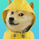จำนวนเข้าชม 91
Before anything, take notes of the script limitations :
1) The script has the setting : "Max_bars_back" set to 390. This is required to use dynamic length and an "internal clock"
*390 is the normal number of bars in a typical stock trading day.

2) The script requires volume data to work correctly
* I use a way to represent volatility that requires ( volume + price movement + time, no sma , no stdev)
Why ? Because:
HIGH-LOW = Fail to get gap information, fail to get volume information
TR /ATR = Fail to get volume information
STDEV = Not very good with dynamic length as it use SMA and fails when length <2

Conclusion: this script is basically limited to stock trading.
If I could set Max_bars_back to 23 400 this would even work better with a 1 second time frame as more data would be entered in the system.

The idea behind the script is that volatility exists in two different formats depending on how you observe the situation.
Remember your physics class? Light can be a wave or a particle depending of the situation.
Volatility seems to share a strange similar property.
Format 1) Instant volatility . (Ex. High - Low, TR )
Format 2) Systemic volatility (Ex. Dynamic ATR with variable length)

I then speculate 3 lines. (on both directions, + or -)
What if all transaction were 50% directional according to the instant volatility
What if all transaction were 100% directional according to the instant volatility
What if all transaction were 200% directional according to the instant volatility
sum(0,5*instant volatility , dynamic time)
sum(instant volatility , dynamic time)
sum(2*instant volatility , dynamic time)

This give you potential signals lines and support/resistance .

I then calculate the real price movement
sum(price movement, dynamic time)

Then you need to compare the real price movement VS if all transaction were 100% directional following the system volatility
dif=(sum price movement/sum volatility )*dynamic time

Voila.

If the movement is directional then it follows the system volatility .
If the movement is not directional then it goes back toward the Zero.

Inspirational image :
https://en.wikipedia.org/wiki/Prism#/media/File:Comparison_refraction_diffraction_spectra.svg
เอกสารเผยแพร่: Version 2 changelog.
1) There was an issue when a bar was missing on the chart.(When no trade happened) Fixed it by using the last known variable.
2) There was an additional similar issue when the volatility was = 0. (variable/0 = error)
3) I've realized that when my instant volatility gets over 1 it has a big impact on the "horizon" of possibility.

At first, I thought it was a bug but it's working has intended.
So, instead of trying to fix that, I've added two customizable lines and a general scale.
Until I can figure out the math behind an autocalibration you might need to calibrate the indicator yourself.

If the "Ratio real position / 100% volatility direction" seems to be stuck close to 0, it means that scale isn't calibrated.
To calibrate the scale, you need to look at yesterday data and change the input "1" to something smaller like 0.1

J. Welles Wilder directional movement is an oscillator between 0 and 100 bounded to a static length.
His idea was great at the time. But I wanted something more dynamic that understand the power of volume.
Something with a dynamic length that still has an idea of what happened at the first minute of the day.

Let's suppose you want to try to predict the future. The farther you need to predict, the harder it gets.
Think like a meteorologist. The closer the date, the more accurate the prediction is.
This is related to the chaos theory or under the common name : Butterfly effect.

Stock movement is about the same.
The price next bar should be close to your current bar.
The price in 280 bars is pretty hard to predict. Especially if you use a static length like Wells Wilder did. Because everything reset after that length of time passed.

So instead of bonding between 0 and 100. Any directional movement index should consider that the horizon of possibility extends as time pass. This is why all resistances and supports lines are diverging from 0.
This is also why using a horizontal line to spot a divergence with this indicator might not be great.
As when stock "move horizontally", it means that it doesn't move. If it doesn't move then what can be diverging?

https://en.wikipedia.org/wiki/Average_di...

## ความคิดเห็นThere is a problem when the stock instant volatility > 1.
Be aware of that. I'm trying to find a solution.
ตอบกลับ